# Test Prep Plan - Take a practice test

Take this practice test to check your existing knowledge of the course material. We'll review your answers and create a Test Prep Plan for you based on your results.
How Test Prep Plans work
1
2Based on your results, we'll create a customized Test Prep Plan just for you!
3Study smarter
Study more effectively: skip concepts you already know and focus on what you still need to learn.

# MTEL Mathematics (Elementary): Circles & Circular Arcs Chapter Exam

Exam Instructions:

Choose your answers to the questions and click 'Next' to see the next set of questions. You can skip questions if you would like and come back to them later with the yellow "Go To First Skipped Question" button. When you have completed the practice exam, a green submit button will appear. Click it to see your results. Good luck!

### Page 1

#### Question 1 1. If angle ACB is 47 degrees, what is the measure of angle AOB?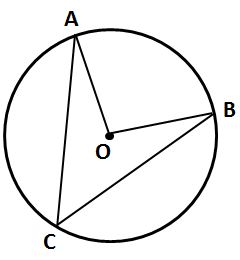#### Question 2 2. If angle ACB is 34 degrees, what is the measure of angle ADB?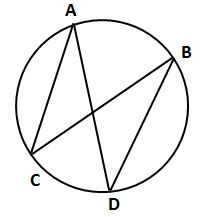#### Question 3 3. In the picture below, if arc BD is 168 degrees and arc BC is 70 degrees, what is the measure of angle BAD?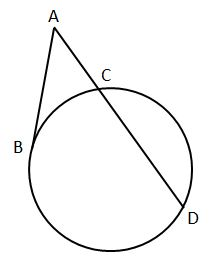#### Question 5 5. In the picture below, if arc AC is 146 degrees and arc BD is 32 degrees, what is the measure of angle AEC?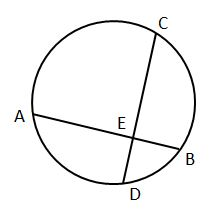### Page 2

#### Question 7 7. What is the line, as shown in the picture, called?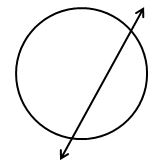### Page 3

#### Question 15 15. The red segment pictured on the circle represents: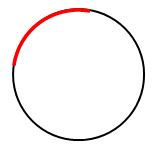### Page 4

#### Question 16 16. Which line is a tangent to the pictured circle?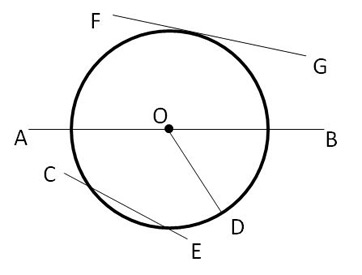#### Question 19 19. What is the area of the following segment? Give your answer to two decimal places.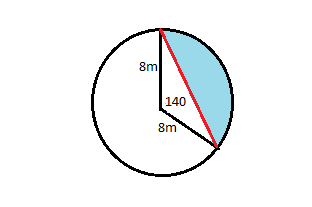### Page 5

#### Question 23 23. In the picture below, if major arc BC is 258 degrees and minor arc BC is 102 degrees, what is the measure of angle BAC?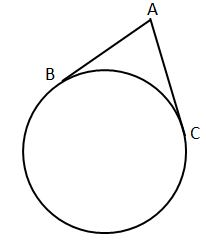#### Question 24 24. In the picture below, if arc AB is 82 degrees, what is the measure of angle BAC?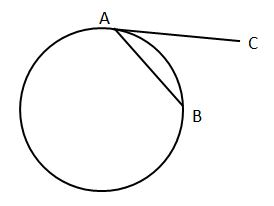#### Question 25 25. If angle OAB is 45 degrees, what is the measure of angle AOB?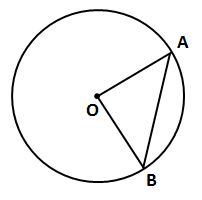### Page 6

#### Question 26 26. If angle AOB is 40 degrees, what is the measure of angle ACB?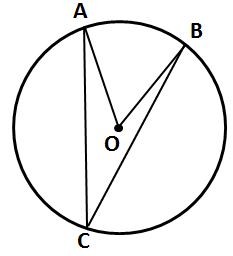#### Question 27 27. In the pictured circle, the measure of angle ACB is 22 degrees and the measure of angle BXD is 120 degrees. What is the measure of angle CAD?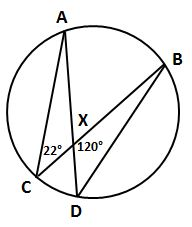#### Question 28 28. If AB and CB are tangent segments, what is the length of CB?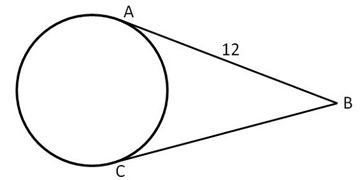#### MTEL Mathematics (Elementary): Circles & Circular Arcs Chapter Exam Instructions

Choose your answers to the questions and click 'Next' to see the next set of questions. You can skip questions if you would like and come back to them later with the yellow "Go To First Skipped Question" button. When you have completed the practice exam, a green submit button will appear. Click it to see your results. Good luck!

Support